# High voltage (>200Vdc) protection and switch circuit to properly bias a MEMS sensor.

#### gino63

Joined Feb 11, 2016
6
Hi guys.
thanks for the hospitality in this forum ! I have a problem to solve....
I have to bias a MEMS sensor that is a condenser of about 30pF (max 100 pF).
To properly operate (in Tx and Rx), the condenser has to be biased using a high DC voltage Vh (min. 50 VDC – max 200 VDC). This sensor needs of a particular scheme of biasing: every terminal of the condenser (X,Y) has to be biased with +Vh or –Vh or GND (alternatively, and for each terminal).
X Y
+Vh GND
-Vh GND
GND +Vh
GND -Vh
GND GND

Furthermore, each terminal of the condenser is attached to a TX/RX circuit, of a Maxim IC (MAX4805A) by two condensers (Tx and In pins). So, the scheme “of principle” can be depicted as in the figure.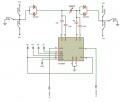Fig. 1

The switches in figure can be of a MAX14802, but the voltage to switch has to be reduced to avoid the break of the MAX14802 analog multiplexer (max 200V). We have to design a “protection” circuit to use MAX14802 in a safe area, with the specified voltage: in fact the voltage is 400VDC at the pins of the open switch, without a protection circuit.
In fig. 2 and 3 there are two “attempts” of circuits design to protect MAX14802, but they suffer of injection charge, as you can see in fig. 4 and 5, respectively.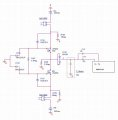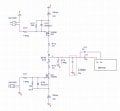Fig. 2 Fig. 3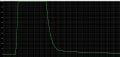Fig. 4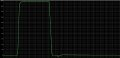Fig. 5

Any help if welcome.

Sincerely
Gino

#### gino63

Joined Feb 11, 2016
6

#### ScottWang

Joined Aug 23, 2012
7,327
Why in the image 2 in series with two 47K resistors in the output, and image 1 there are no 47K resistors?

How is the voltage of D02cz13?

Fig. 4 related to which test point and which image, Fig. 5 related to which test point and which image?

If the discharge is in the image 1 then it should reduce the value of R167 and maybe could adds a quick discharge circuit, because it was Ciss of mosfet caused that.

How is the frequency of Tx, Rx?

#### gino63

Joined Feb 11, 2016
6
Why in the image 2 in series with two 47K resistors in the output, and image 1 there are no 47K resistors?

How is the voltage of D02cz13?

Fig. 4 related to which test point and which image, Fig. 5 related to which test point and which image?

If the discharge is in the image 1 then it should reduce the value of R167 and maybe could adds a quick discharge circuit, because it was Ciss of mosfet caused that.

How is the frequency of Tx, Rx?
1) In the second circuit (fig. 3) there are 2 resistors (47k) to limit the current. In the first one it's not necessary, in my opinion.
2) The zener diode is 13V.
3) Fig. 4 is related to the circuit in fig, 2 and the test point is on the sensor (C_sensor)
4) Fig. 5 is related to the circuit in fig. 3 and the test point is the same (C_sensor)
5) Ok, reducing the value of R167 the discharge is more quick, but increasing the current....
6) The frequency of Rx/Tx is in the range 6-18 MHz

#### ScottWang

Joined Aug 23, 2012
7,327
1) In the second circuit (fig. 3) there are 2 resistors (47k) to limit the current. In the first one it's not necessary, in my opinion.
If they are using the same load, why one circuit needed and another one doesn't need?

5) Ok, reducing the value of R167 the discharge is more quick, but increasing the current.
What you think where will be increasing the current(D)?
When the Vgs over 10V then D(n type) output and S will be as a switch, so increasing the Vgs won't increasing the D current, if the values of Rg(R167) too high then it will be affecting the discharge time, VP0550 - Ciss 40pf(typ), 70pf(max).

I saw a circuit used a pnp to discharge the current for Vgs.

I_R167 = (200V - D134-D133)/R167
= (200V - 13V -0.7V)/3.3M
= 186.3V/3.3M
= 56.5uA
The current can be lower as 1mA.
R167 = 186.3V/1mA
= 186.3K, you can choose 190K or 200K or 220K

#### gino63

Joined Feb 11, 2016
6
If they are using the same load, why one circuit needed and another one doesn't need?

What you think where will be increasing the current(D)?
When the Vgs over 10V then D(n type) output and S will be as a switch, so increasing the Vgs won't increasing the D current, if the values of Rg(R167) too high then it will be affecting the discharge time, VP0550 - Ciss 40pf(typ), 70pf(max).

I saw a circuit used a pnp to discharge the current for Vgs.

I_R167 = (200V - D134-D133)/R167
= (200V - 13V -0.7V)/3.3M
= 186.3V/3.3M
= 56.5uA
The current can be lower as 1mA.
R167 = 186.3V/1mA
= 186.3K, you can choose 190K or 200K or 220K
I am agree with you about R167, but the problem is that I have many of these sensors, and if I increase the discharge current from 56uA to 1mA the entire board needs a large current @ Vh (240 sensors = 240mA = 48W (@200V, too much).

Another solution is to switch "ON" M31 for a small time (1-2 us) to discharge the injection ....
What do you think ?
I am working on this now....
TY
Gino

#### ScottWang

Joined Aug 23, 2012
7,327

Another common method is to using the 1n4148 diode in parallel with R170 and R171, the diode with R170 is negative at the right side, and the diode with R171 is negative at the left side.

If you trying to using the software to do the discharge time then you could try it.

#### gino63

Joined Feb 11, 2016
6

Another common method is to using the 1n4148 diode in parallel with R170 and R171, the diode with R170 is negative at the right side, and the diode with R171 is negative at the left side.

If you trying to using the software to do the discharge time then you could try it.
Thanks of the comment: tomorrow morning I'll try with the diodes.
I have some interesting results using the "software". I'll show tomorrow. The circuit (fig. 2) is very interesting, because exhibits a "virtual ground" for large signals and not for small signals. And this characteristic is very important in this application. The background is a problem with the timing of the switches: if there are some differences between the "turn-on/off" time, the circuit has some problems. I'll be more clear tomorrow.
For the moment, thank you.
Gino

#### RichardO

Joined May 4, 2013
2,270
This is how Polaroid/TI did it long ago in instant cameras. Look at the very end of the document.

#### Attachments

• 695.2 KB Views: 9

#### gino63

Joined Feb 11, 2016
6
Dear all,
the entire circuit to protect the switches of MAX14802 is depicted in fig. 1.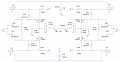Fig. 1

The two switches on the right are open, so the circuit on the right is "substantially" off, but the relative pin of the condenser is at "virtual ground". Now, in a first simulation, we close the switch S2 (on the top-left): all the other switches are open. So, in the top circuit on the left is applied +200 Vdc. The differential voltage at C_sensor is in fig.2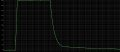Fig. 2

In a successive simulation, when the switch S2 will be opened (@400us), the switch S1 (bottom-left) is closed for 2us. The differential voltage at C_sensor is in fig. 3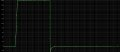Fig. 3

As you can see, the injection charge was shunted by this circuit very well. The current used by the circuit, in this example is about 130-140uA that is very nice. The problem is that the circuit is very dependent by the difference in timing of the switches: if there is a dispersion in the characteristic of the switches, this is reflected in the characteristics of the entire circuit.
If someone want a negative bias on the left-terminal of the sensor, use S1 and S2 on the contrary. If someone want a bias on the right-terminal of the sensor, has to be used the switches S3 and S4, leaving open S1 and S2.

Guys, what do you think ?
TY
Gino